# Python 函数3000字使用总结

Python实战社群

Java实战社群• Python 函数专题

• 基础部分

• 1 函数组成

• 2 引用传参

• 3 默认参数与关键字参数

• 4 可变参数

• 5 内置函数

• 进阶部分

• 6 偏函数

• 7 递归函数

• 8 匿名函数

• 9 高阶函数

• 10 嵌套函数

• 总结

## Python 函数专题

Python提供了printsortedmaxmap等内置函数，但我们也可以创建自己的函数，称为用户定义函数。

## 基础部分

### 1 函数组成

def foo(nums):
""" 返回偶数序列"""
evens = []
for num in nums:
if num%2==0:
evens.append(num)
return evens


• 函数名：foo

• 函数形参：nums

• :: 函数体的控制字符，作用类似JavaC++的一对{}

• 缩进：一般为4个字符

• """：为函数添加注释

• return: 函数返回值

### 2 引用传参

foo([10,2,5,4])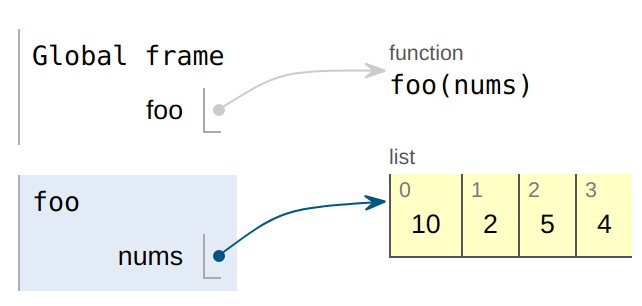def myFun(x):
x = 20


lst = [10, 11, 12, 13, 14, 15]
myFun(lst)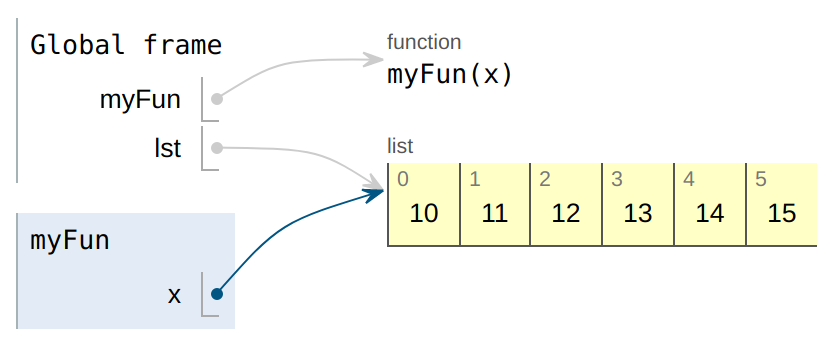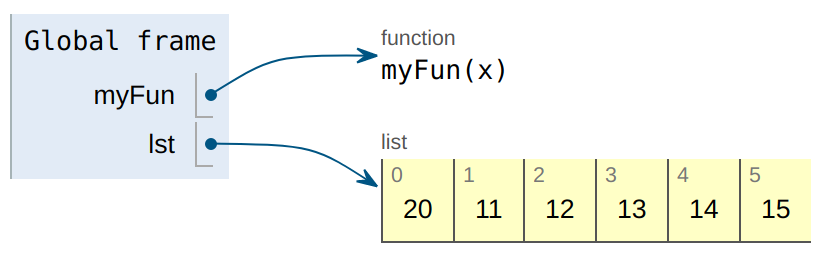def myFun(x):
x = [20, 30, 40]
x = 0


lst = [10, 11, 12, 13, 14, 15]
myFun(lst)


x 被传参后初始指向lst，如下所示：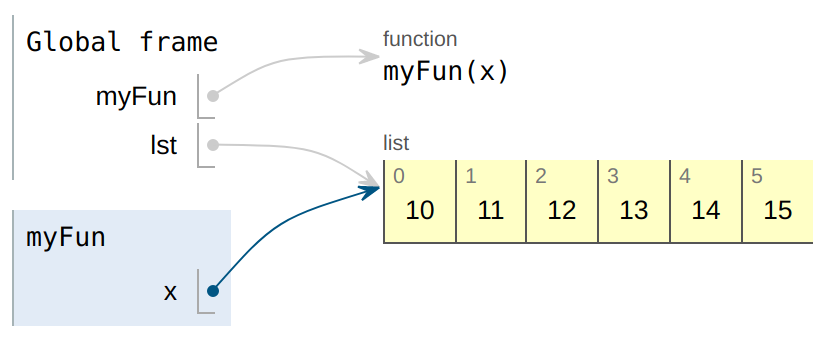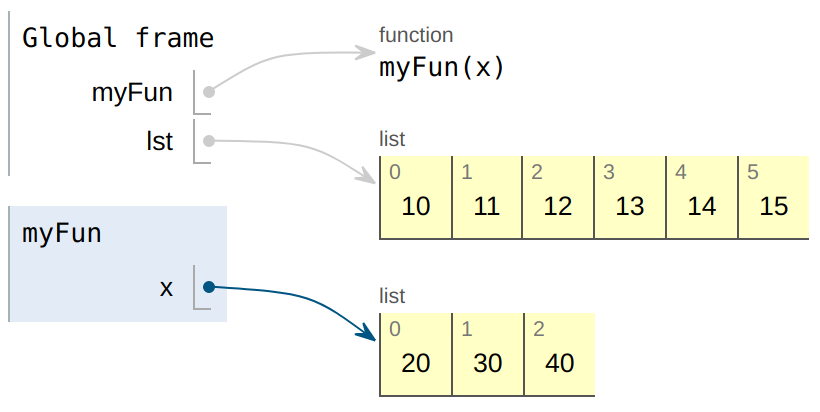### 3 默认参数与关键字参数

Python函数的参数，可以有初始默认值，在调用时如果不赋值，则取值为默认值，如下例子：

def foo(length,width,height=1.0):
return length*width*height


r = foo(1.2,2.0)
print(r) # 2.4


def foo(length,width,height=1.0): # height是默认参数
return length*width*height

foo(width=2.0,length=1.2) #确定这种调用后才确定width和length是关键字参数


### 4 可变参数

JavaC++在解决同一个函数但参数个数不同时，会使用函数重载的方法。Python使用可变参数的方法，非常灵活。

def foo(length,*others):
s = length
for para in others:
s *= para
return s


foo(1.2,2.0,1.0) # 2.4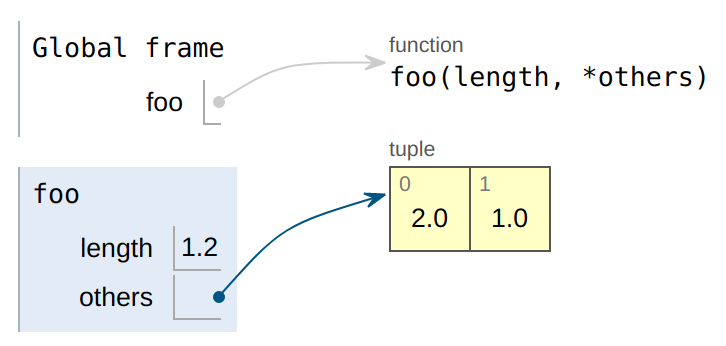foo(1.2) # 1.2


### 5 内置函数

#### pow

pow(2,3)


pow(2,3,5) # 3


#### max,min

max,min用来求解最大最小值，实现relu函数：

def relu(x):
return max(x,0)


#### sorted

sorted函数完成对象排序，它能接收一个指定排序规则的函数，完成定制排序。如下，根据字典值绝对值从小到大排序：

d = {'a':0,'b':-2,'c':1}
dr = sorted(d.items(),key=lambda x:abs(x))
print(dr) # [('a', 0), ('c', 1), ('b', -2)]


## 进阶部分

Python有一个专门操作函数的模块：functools，能实现一些关于函数的特殊操作。

### 6 偏函数

int('1010',base=2)


def int2(s):
return int(s,base=2)


from functools import partial

intp = partial(int,base=2)


intp('10',base=16) # 16


### 7 递归函数

def reverseStr(s):
if not s:
return s
return reverseStr(s[1:])+s

print(reverseStr('nohtyp')) # python


reverseStr函数里面又调用了函数reverseStr，所以它是递归函数。

### 8 匿名函数

lambda 形参列表: 含有形参列表的表达式


lambda x,y: x+y


def f(x,y):
return x+y


lambda函数常做为max,sorted,map,filter等函数的key参数。

### 9 高阶函数

def f(g):
g()


Python 中经常会遇到高阶函数，今天介绍几个内置的常用的高阶函数。

#### map

map 函数第一个参数为函数，它作用于列表中每个的元素。

m = map(lambda s: s.capitalize(), ['python','Very','BEAUTIFUL'])
print(list(m))


['Python', 'Very', 'Beautiful']


#### reduce

reduce 高阶函数实现化简列表，它实现的效果如下：

from functools import reduce

def f(x,y):
return x+y

r = reduce(f, [1,3,2,4])
print(r) # 10


### 10 嵌套函数

nums = [3,9,20,None,None,15,7]，转化为下面二叉树: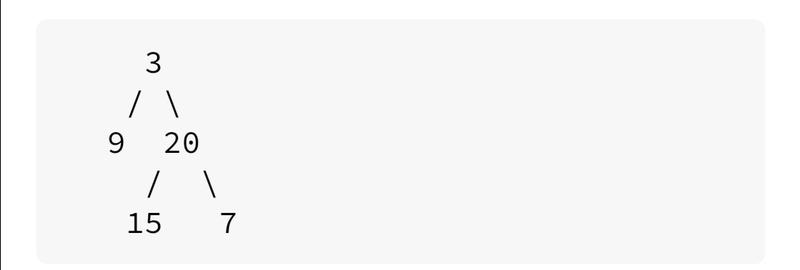class TreeNode:
def __init__(self, x):
self.val = x
self.left = None
self.right = None


if index >= len(nums) or nums[index] is None:
return None


def list_to_binarytree(nums):
def level(index):
if index >= len(nums) or nums[index] is None:
return None

root = TreeNode(nums[index])
root.left = level(2 * index + 1)
root.right = level(2 * index + 2)
return root

return level(0)

binary_tree = list_to_binarytree([3,9,20,None,None,15,7])


## 总结

• Python 函数专题

• 基础部分

• 1 函数组成

• 2 引用传参

• 3 默认参数与关键字参数

• 4 可变参数

• 5 内置函数

• 进阶部分

• 6 偏函数

• 7 递归函数

• 8 匿名函数

• 9 高阶函数

• 10 嵌套函数

• 总结程序员专栏 扫码关注填加客服 长按识别下方二维码进群


10-08176
02-01303508-0957
11-23151
04-231万+
09-197969
08-103958
09-061741
04-024185
09-202535